Q-Chem 5.0 User’s Manual

# 4.7 SCF Calculations Based on GEN_SCFMAN

## 4.7.1 Overview

GEN_SCFMAN is an object-oriented SCF job manager in Q-Chem developed by E. J. Sundstrom, P. R. Horn and many other co-workers. Up till now, it has been able to support most of the basic features of the old SCF routines (e.g. restricted, unrestricted and restricted open-shell Hartree-Fock/KS-DFT calculations). Besides that, many absent features in the old SCF code are available in GEN_SCFMAN, mainly including

• Addition of several useful SCF algorithms and support of user-specified hybrid algorithm.

• More general and user-friendly internal stability analysis and automatic correction for the energy minimum.

• Support of several unconventional orbital types.

A full list of orbital types supported in GEN_SCFMAN:

• The basic ones that are supported in the old SCF code: restricted (R), unrestricted (U), restricted open-shell (RO).

• Open-shell singlet ROSCF (OS_RO, see the “ROKS" method documented in Section 6.5).

• Generalized Hartree-Fock method (G), i.e., each MO is associated with bothand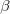spin components.

• The use of complex orbitals (with Hartree-Fock only): restricted (CR), unrestricted (CU), and generalized (CG).

A list of useful SCF algorithms available in GEN_SCFMAN (each entry stands for a method that the $rem variable SCF_ALGORITHM can be set to): Methods that are based on extrapolation/interpolation: • DIIS (see Section 4.6.3, available for all orbital types). • ADIIS (the Accelerated DIIS algorithm developed by Hu et al. , available for R and U only). Methods that make use of orbital gradient: • DM (re-implemented as simple steepest descent with line search, available for all orbital types). • GDM (see Section 4.6.4, available for all orbital types). • GDM_LS (it is essentially a preconditioned (using orbital energy differences as the preconditioner) L-BFGS algorithm with line search, available for R, U, RO and OS_RO). Methods that require orbital Hessian: • NEWTON_CG/NEWTON_MINRES (solve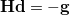for the update direction with CG/MINRES solvers). • SF_NEWTON_CG (the “saddle-free" version of NEWTON_CG). The analytical orbital Hessian is available for R/U/RO/G/CR unless special density functionals (e.g. those containing VV10) are used, while the use of finite-difference Hessian is available for all orbital types by setting FD_MAT_VEC_PROD = TRUE. Besides these, the Maximum-Overlap Method (MOM) for excited states is also available. We note that the RCA algorithm is not available in GEN_SCFMAN yet. Nevertheless, the performance of the ADIIS algorithm should be similar to it. Since the code in GEN_SCFMAN is highly modular, the availability of different SCF algorithms to different SCF (orbital) types is largely extended in general. For example, the old ROSCF implementation requires the use of the GWH guess and the GDM algorithm exclusively. Such a limitation has been eliminated in GEN_SCFMAN based RO calculations. The other aspects of an SCF calculation, such as the SCF guess, the use of efficient algorithms to construct the Fock matrix like occ-RI-K (see Section 4.8.11), would not be affected by the use of GEN_SCFMAN. Also, using GEN_SCFMAN would not make any difference to the post-SCF procedures such as correlated methods, excited state calculations and evaluation of molecular properties. It should be noted that many special features (e.g. dual-basis SCF, CDFT, etc.) based on Q-Chem’s old SCF code are not supported in GEN_SCFMAN yet. They will gradually become available in the future. ## 4.7.2 Basic GEN_SCFMAN Job Control For standard R/U/RO calculations using GEN_SCFMAN, the setup of the input is basically identical to whatever the old SCF code requires, except that we need to set GEN_SCFMAN = TRUE. GEN_SCFMAN  Use GEN_SCFMAN for the present SCF calculation. TYPE:  BOOLEAN DEFAULT:  FALSE OPTIONS:  FALSE Use the old SCF code. TRUE Use GEN_SCFMAN. RECOMMENDATION:  Setting to TRUE when features in GEN_SCFMAN are needed. Example 4.54 Restricted SCF calculation using GEN_SCFMAN molecule 0 1 O -1.551007 -0.114520 0.000000 H -1.934259 0.762503 0.000000 H -0.599677 0.040712 0.000000$end

$rem JOBTYPE sp METHOD wB97X-V BASIS def2-tzvpd GEN_SCFMAN true UNRESTRICTED false SCF_ALGORITHM diis SCF_CONVERGENCE 8 THRESH 14 SYMMETRY false SYM_IGNORE true SCF_FINAL_PRINT 1$end


In the up-to-date GEN_SCFMAN, RO calculations are triggered in the same way as in the old SCF routines (when UNRESTRICTED = FALSE and the multiplicity of the system is greater than 1). Also, setting $rem variable ROSCF = TRUE forces GEN_SCFMAN to run an RO calculation. ROSCF  Run an ROSCF calculation with GEN_SCFMAN. TYPE:  BOOLEAN DEFAULT:  FALSE OPTIONS:  FALSE Let Q-Chem automatically decide if RO is needed. TRUE Run an ROSCF calculation forcefully. RECOMMENDATION:  No need to set this rem for standard calculations. Example 4.55 Restricted open-shell SCF calculation using GEN_SCFMAN. The DIIS algorithm is employed. $molecule
0 2
C1
H1  C1  1.09267
H2  C1  1.09267  H1  107.60335
H3  C1  1.09267  H2  107.60335  H1  115.692
$end$rem
JOBTYPE           sp
METHOD            wB97X-D
BASIS             6-31+G*
GEN_SCFMAN        true
UNRESTRICTED      false  !this triggers the RO calculation
MAX_SCF_CYCLES    100
SCF_ALGORITHM     diis
SCF_CONVERGENCE   8
THRESH            14
SYMMETRY          false
SYM_IGNORE        true
SCF_FINAL_PRINT   1
$end  The calculations using other more special orbital types are controlled by the following$rem variables (they are not effective unless GEN_SCFMAN = TRUE):

OS_ROSCF
 Run an open-shell singlet ROSCF calculation with GEN_SCFMAN.

TYPE:
 BOOLEAN

DEFAULT:
 FALSE

OPTIONS:
 TRUE OS_ROSCF calculation is performed. FALSE Do not run OS_ROSCF (it will run a close-shell RSCF calculation instead).

RECOMMENDATION:
 Set to TRUE if desired.

GHF
 Run a generalized Hartree-Fock calculation with GEN_SCFMAN.

TYPE:
 BOOLEAN

DEFAULT:
 FALSE

OPTIONS:
 TRUE Run a GHF calculation. FALSE Do not use GHF.

RECOMMENDATION:
 Set to TRUE if desired.

COMPLEX
 Run an SCF calculation with complex MOs using GEN_SCFMAN.

TYPE:
 BOOLEAN

DEFAULT:
 FALSE

OPTIONS:
 TRUE Use complex orbitals. FALSE Use real orbitals.

RECOMMENDATION:
 Set to TRUE if desired.

COMPLEX_MIX
 Mix a certain percentage of the real part of the HOMO to the imaginary part of the LUMO.

TYPE:
 INTEGER

DEFAULT:
 0

OPTIONS:
 0–100 The mix angle =COMPLEX_MIX/100.

RECOMMENDATION:
 It may help find the stable complex solution (similar idea as SCF_GUESS_MIX).

Example 4.56  Restricted open-shell singlet ROSCF calculation for the first excited state of formaldehyde using GEN_SCFMAN. The first job provides the guess orbitals through a restricted SCF calculation.

$molecule 0 1 H -0.940372 0.000000 1.268098 H 0.940372 0.000000 1.268098 C 0.000000 0.000000 0.682557 O 0.000000 0.000000 -0.518752$end

$rem GEN_SCFMAN true METHOD wb97x-d BASIS def2-svpd THRESH 14 SCF_CONVERGENCE 9 SYM_IGNORE true$end

@@@

$molecule read$end

$rem JOBTYPE sp METHOD wb97x-d BASIS def2-svpd GEN_SCFMAN true OS_ROSCF true THRESH 14 SCF_CONVERGENCE 9 SCF_ALGORITHM diis SYM_IGNORE true SCF_GUESS read$end


## 4.7.3 User-Customized Hybrid SCF Algorithm

It is often the case that a single algorithm is not able to guarantee SCF convergence. Meanwhile, some SCF algorithms (e.g. ADIIS) can accelerate convergence at the beginning of an SCF calculation but becomes less efficient near the convergence. While a few hybrid algorithms (DIIS_GDM, RCA_DIIS) have been enabled in Q-Chem’s original SCF implementation, in GEN_SCFMAN, we seek for a more flexible setup for the use of multiple SCF algorithms so that users can have a more precise control on the SCF procedure. With the current implementation, at most four distinct algorithms (usually more than enough) can be employed in one single SCF calculation based on GEN_SCFMAN, and the basic job control is as follows:

GEN_SCFMAN_HYBRID_ALGO
 Use multiple algorithms in an SCF calculation based on GEN_SCFMAN.

TYPE:
 BOOLEAN

DEFAULT:
 FALSE

OPTIONS:
 FALSE Use a single SCF algorithm (given by SCF_ALGORITHM). TRUE Use multiple SCF algorithms (to be specified).

RECOMMENDATION:
 Set it to TRUE when the use of more than one algorithm is desired.

GEN_SCFMAN_ALGO_1
 The first algorithm to be used in a hybrid-algorithm calculation.

TYPE:
 STRING

DEFAULT:
 0

OPTIONS:
 All the available SCF_ALGORITHM options, including the GEN_SCFMAN additions (Section 4.7.1).

RECOMMENDATION:
 None

GEN_SCFMAN_ITER_1
 Maximum number of iterations given to the first algorithm. If used up, switch to the next algorithm.

TYPE:
 INTEGER

DEFAULT:
 50

OPTIONS:
 User-defined

RECOMMENDATION:
 None

GEN_SCFMAN_CONV_1
 The convergence criterion given to the first algorithm. If reached, switch to the next algorithm.

TYPE:
 INTEGER

DEFAULT:
 0

OPTIONS:10RECOMMENDATION:
 None

Note: $rem variables GEN_SCFMAN_ALGO_X, GEN_SCFMAN_ITER_X, GEN_SCFMAN_CONV_X (X = 2, 3, 4) are defined and used in a similar way. Example 4.57 B3LYP/3-21G calculation for a cadmium-imidazole complex using the ADIIS-DIIS algorithm (an example from Ref. Hu:2010). Due to the poor quality of the CORE guess, using a single algorithm such as DIIS or GDM fails to converge. $molecule
2 1
Cd     0.000000     0.000000     0.000000
N      0.000000     0.000000    -2.260001
N     -0.685444     0.000000    -4.348035
C      0.676053     0.000000    -4.385069
C      1.085240     0.000000    -3.091231
C     -1.044752     0.000000    -3.060220
H      1.231530     0.000000    -5.300759
H      2.088641     0.000000    -2.711077
H     -2.068750     0.000000    -2.726515
H     -1.313170     0.000000    -5.174718
$end$rem
JOBTYPE                  SP
EXCHANGE                 B3LYP
BASIS                    3-21g
UNRESTRICTED             FALSE
SYMMETRY                 FALSE
SYM_IGNORE               TRUE
THRESH                   14
SCF_GUESS                CORE
GEN_SCFMAN               TRUE
GEN_SCFMAN_HYBRID_ALGO   TRUE
GEN_SCFMAN_CONV_1        3  !switch to DIIS when error < 1E-3
GEN_SCFMAN_ITER_1        50
GEN_SCFMAN_ALGO_2        DIIS
GEN_SCFMAN_CONV_2        8
GEN_SCFMAN_ITER_2        50
$end  ## 4.7.4 Internal Stability Analysis and Automated Correction for Energy Minima Since an SCF algorithm searches for stationary points where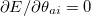, chances always exist that an unstable solution is found, for which the orbital Hessian is not positive-definite. Based on our experience, even for very simple data set such as the G2 atomization energies, using the default algorithm (DIIS) produces unstable solutions for quite a few species (even for single atoms with some density functionals). In such scenarios, failure to check the internal stability of SCF solutions can result in flawed benchmark results. Although in general the use of gradient-based algorithms such as GDM is more likely to locate the true minimum, it still cannot entirely eliminate the possibility for an unstable solution to appear. The original STABILITY_ANALYSIS method for calculations using the old SCF code has the following limitations: • It is only available for restricted (close-shell) and unrestricted SCF calculations. • It requires the analytical orbital Hessian of the wave function energy. • The calculation terminates after the corrected MOs are generated, and a second job is needed to read in these orbitals and run another SCF calculation. The implementation of internal stability analysis in GEN_SCFMAN overcomes almost all these shortcomings. Its availability has been extended to all the implemented orbital types. As in the old code, when the analytical Hessian of the given orbital type and theory (e.g. RO/B3LYP) is available, it computes matrix-vector products requested by the Davidson algorithm  analytically. If the analytical Hessian is not available, user can still run stability analysis by using the finite-difference matrix-vector product technique developed by Sharada et al. which requires the gradient (related to the Fock matrix) only: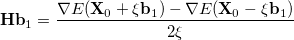(4.92) where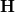is the Hessian matrix,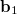is a trial vector,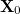stands for the current stationary point, andis the finite step size. With this method, internal stability analysis is available for all the implemented orbital types in GEN_SCFMAN. It should be noted that since the second derivative of NLC functionals such as VV10 is not available in Q-Chem, this finite-difference method will be used by default for the evaluation of Hessian-vector products, while the old STABILITY_ANALYSIS method is not capable of handling functionals that contain NLC. Meanwhile, GEN_SCFMAN allows multiple SCF calculations + stability analyses to be performed in a single job so that it can make use of the corrected MOs and locate the true minimum automatically. As in the old implementation, the MOs are displaced along the direction of the lowest-energy eigenvector (with line search) if an SCF solution is found to be unstable. A new SCF calculation that reads in these corrected MOs as initial guess will be launched automatically if INTERNAL_STABILITY_ITER > 0. Such macro-loops will keep going until a stable solution is reached. INTERNAL_STABILITY  Perform internal stability analysis in GEN_SCFMAN. TYPE:  BOOLEAN DEFAULT:  FALSE OPTIONS:  FALSE Do not perform internal stability analysis after convergence. TRUE Perform internal stability analysis and generate the corrected MOs. RECOMMENDATION:  Turn it on when the SCF solution is prone to unstable solutions, especially for open-shell species. FD_MAT_VEC_PROD  Compute Hessian-vector product using the finite difference technique. TYPE:  BOOLEAN DEFAULT:  FALSE (TRUE when the employed functional contains NLC) OPTIONS:  FALSE Compute Hessian-vector product analytically. TRUE Use finite difference to compute Hessian-vector product. RECOMMENDATION:  Set it to TRUE when analytical Hessian is not available. Note: For simple R and U calculations, it can always be set to FALSE, which indicates that only the NLC part will be computed with finite difference. INTERNAL_STABILITY_ITER  Maximum number of new SCF calculations permitted after the first stability analysis is performed. TYPE:  INTEGER DEFAULT:  0 (automatically set to 1 if INTERNAL_STABILITY = TRUE) OPTIONS:new SCF calculations permitted. RECOMMENDATION:  Give a larger number if 1 is not enough (still unstable). INTERNAL_STABILITY_DAVIDSON_ITER  Maximum number of Davidson iterations allowed in one stability analysis. TYPE:  INTEGER DEFAULT:  50 OPTIONS:Perform up toDavidson iterations. RECOMMENDATION:  Use the default. INTERNAL_STABILITY_CONV  Convergence criterion for the Davidson solver (for the lowest eigenvalues). TYPE:  INTEGER DEFAULT:  4 (3 when FD_MAT_ON_VECS = TRUE) OPTIONS:Terminate Davidson iterations when the norm of the residual vector is below 10. RECOMMENDATION:  Use the default. INTERNAL_STABILITY_ROOTS  Number of lowest Hessian eigenvalues to solve for. TYPE:  INTEGER DEFAULT:  2 OPTIONS:Solve forlowest eigenvalues. RECOMMENDATION:  Use the default. Example 4.58 Unrestricted SCF calculation of triplet Busing B97M-V/6-31g with the GDM algorithm. A displacement is performed when the first solution is characterized as a saddle point, and the second SCF gives a stable solution. $molecule
0 3
b
b 1 R

R = 1.587553
$end$rem
JOBTYPE              sp
METHOD               b97m-v
BASIS                6-31g
GEN_SCFMAN           true
UNRESTRICTED         true
THRESH               14
SYMMETRY             false
SYM_IGNORE           true
SCF_FINAL_PRINT      1
SCF_ALGORITHM        gdm
SCF_CONVERGENCE      9
INTERNAL_STABILITY   true  !turn on internal stability analysis
FD_MAT_VEC_PROD      false !use finite-diff for the vv10 part only
\$end